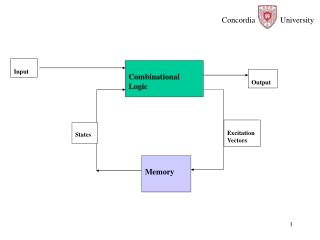DownloadDownload PresentationExcitation Vectors

# Excitation Vectors

Download Presentation## Excitation Vectors

- - - - - - - - - - - - - - - - - - - - - - - - - - - E N D - - - - - - - - - - - - - - - - - - - - - - - - - - -
##### Presentation Transcript

1. Input Combinational Logic Output Excitation Vectors States Memory

2. RS Latch Q+ = S + R’ Q Two Problems: R=S= 1 Not allowed, Data is transparent

3. Q D CLK The D Latch Q+ = D Problem: Level sensitive

4. JK Latch : Universal, Level sensitive, Timing Constraints due to feed back.

5. Master Slave Flip Flop Edge sensitive,Set up and Hold time

6. Master Slave Flip Flop Edge sensitive,-Falling Edge Set Up and Hold Time constraints Path to setup data Master Slave Q Q Q D D D D D Latch Latch Q C C C Path to hold data D C Q

7. Edge triggered Flip Flop: Set up and Hold time Constraints

8. Edge Triggered, D Flip Flop, With Reset NAND1 S Q NAND2 NAND5 clk R NAND3 NAND6 D NAND4 reset Active Low

9. Edge Triggered, D Flip Flop When CLK=0 NAND1 S 1 0 Q NAND2 NAND5 Clk=0 0 R 1 NAND3 NAND6 D NAND4 reset Active Low

10. NAND1 S Q NAND2 NAND5 clk R NAND3 NAND6 D NAND4 reset When CLK changes from 0 to 1 Case1, D=0: tsetup= t4, thold=t3 Path for hold Path for set up

11. NAND1 S Q NAND2 NAND5 clk R NAND3 NAND6 D NAND4 reset When CLK changes from 0 to 1 Case2, D=1 tsetup=t4 + t1 thold= t2 Path to set up Path to hold

12. NAND1 S Q NAND2 NAND5 clk R NAND3 NAND6 D NAND4 reset When CLK changes from 0 to 1 Case1, D=0: tsetup= t4, thold=t3 Case2, D=1 tsetup=t4 + t1 thold= t2

13. D Flip Flop

14. Q+ = JQ’ + K’Q

15. J T T Q K CLK CLK Q T-Flip Flop Q+ = JQ’ + K’Q J=K=T Q+ =TQ’ + T’ Q T=1 Q+ = Q’ T=0 Q+ = Q

16. Q D D T Q T CLK CLK CLK Q T-Flip Flop Q+ = JQ’ + K’Q J=K=T Q+ =TQ’ + T’ Q T=1 Q+ = Q’ T=0 Q+ = Q